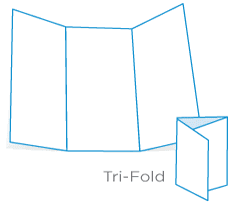## Posts

Showing posts from February, 2019

### Pocket maths: folding halves into thirdsPt En < change language I have folded a piece of paper in half hundreds of times in my life. And probably so did you. Folding a piece of paper in half is fairly easy: just bend the piece of paper until the corners meet, and then crease. That is it. And with this method one can also fold a piece of paper in $4$, in $8$, etc. We just have to successively divide the sections of the paper in half. But what if we wanted to fold a piece of paper into thirds, as in the picture above? Some people are good at doing that, but they don't really measure anything: they just do it approximately by looking at the paper and folding where it seems about right. I guess it goes without saying, but mathematicians don't like things to be "about right", they want them right... and even though I wasn't a mathematician, when I was a child I thought that maybe there was a way for me to successively fold different parts of the paper in half, until one of the creases w

### Problem #14 - the sum of the parts!

Pt En < change language I got the idea for this problem after attending the Recreational Maths Colloquium VI , where I heard about the Thue-Morse sequence for the first time in my life. Problem statement : given the integers $0, 1, \cdots, 15$, find a way to split them into two sets $A$ and $B$ such that $$\sum_{a \in A} a^k = \sum_{b \in B} b^k,\quad k = 0,\cdots,3$$ where we define $0^0 = 1$. Solution : this problem is trivial if we know what we are looking for; if not, I would assume this can only be solved by very resilient people or by those with the help of a computer. Notice that the restriction above, for $k = 0$, just tells us that the sets $A$ and $B$ have the same number of elements. Consider this sequence of length $16$: $$ABBABAABBAABABBA$$ If we identify the sequence with the first $16$ non-negative integers, we get our two sets: $$A = \{0, 3, 5, 6, 9, 10, 12, 15\}$$ $$B = \{1, 2, 4, 7, 8, 11, 13, 14\}$$ Some calculations suffice to show that this i